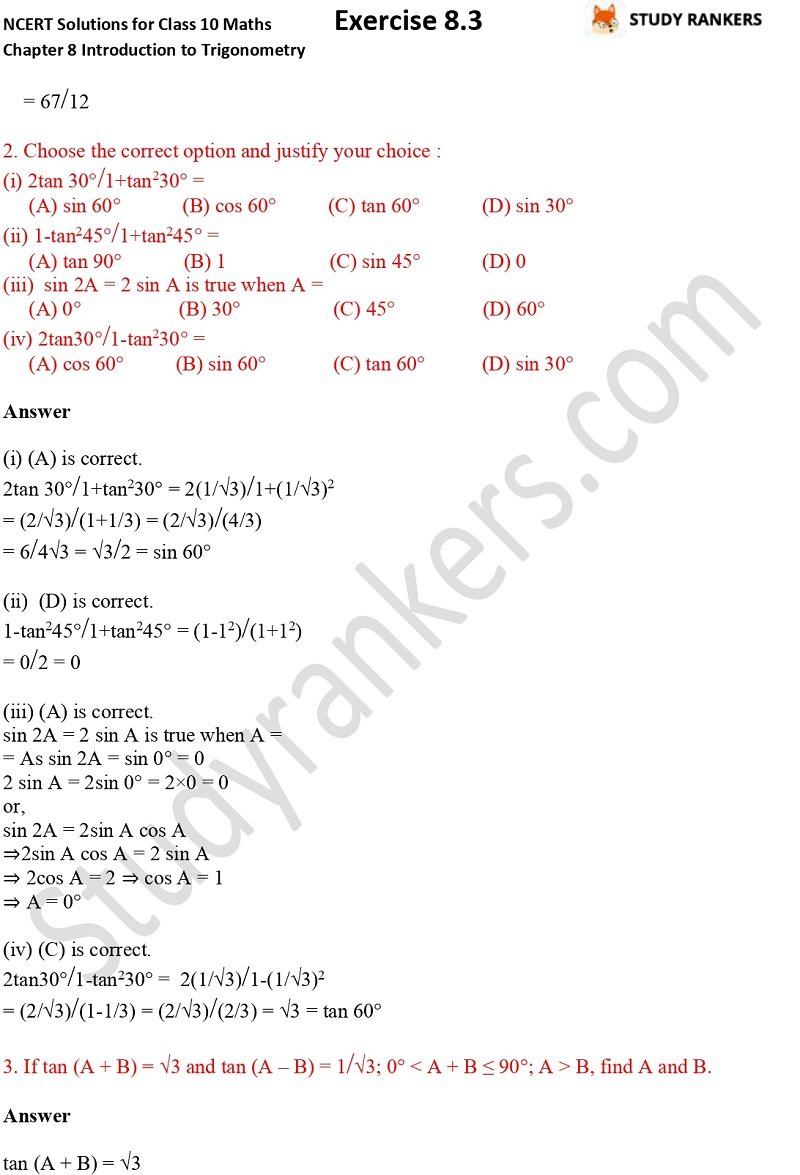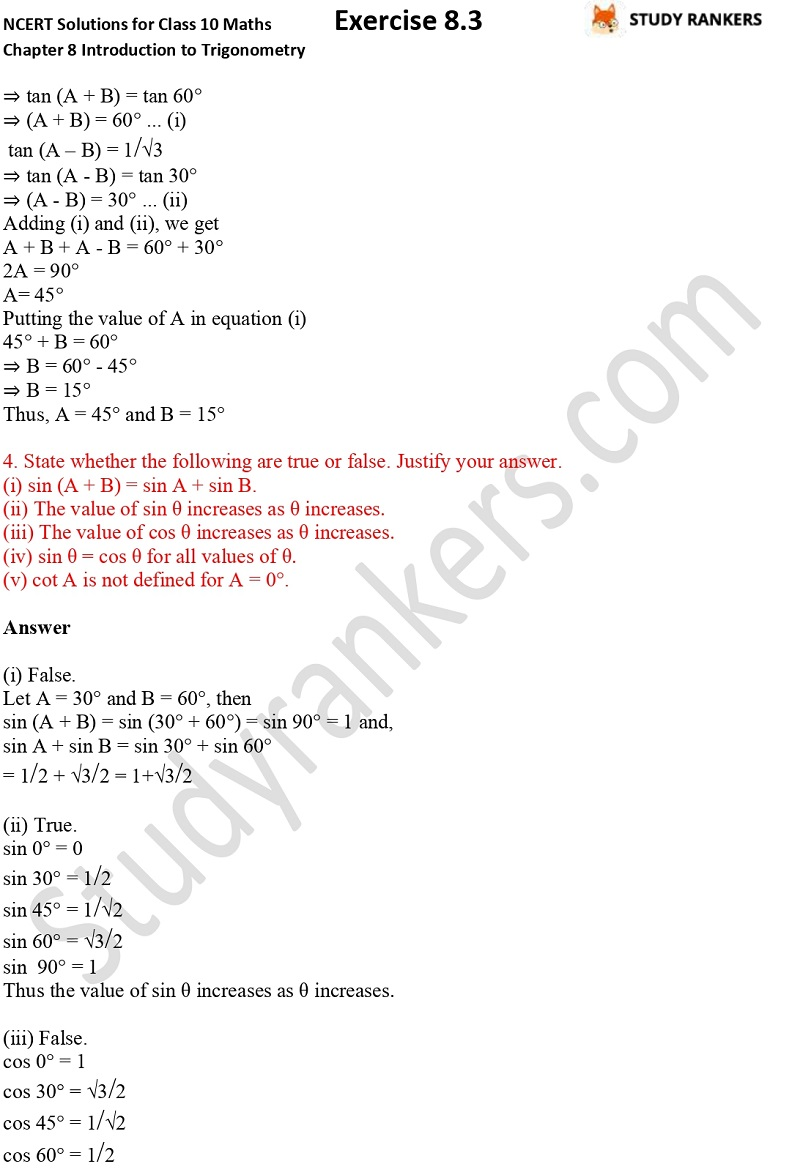>

## NCERT Solutions for Class 10 Maths Chapter 8 Introduction To Trigonometry Exercise 8.2

If you want accurate Chapter 8 Introduction To Trigonometry Exercise 8.2 Class 10 Maths NCERT Solutions then you're at right place. Here you will find step by step solutions of every problems so you can excel in examinations. It will helpful for you in framing own answers and completing school task in time. NCERT Solutions are very important in building basic concepts before going for advance books. These solutions are updated as per the latest marking scheme released by CBSE.

There are three questions which has variety questions such as If tan (A + B) = √3 and tan (A – B) = 1/√3; 0° < A + B ≤ 90°; A > B, find A and B.